# How to Do a vLookup in Python using pandas

Vlookup is essentially used for vertically arranged data. Vlookup is an operation used to merge 2 different data tables based on some condition where there must be at least 1 common attribute(column) between the two tables. After performing this operation we get a table consisting of all the data from both the tables for which the data is matched.

We can use `merge()` function to perform Vlookup in pandas. The merge function does the same job as the Join in SQL We can perform the merge operation with respect to table 1 or table 2.There can be different ways of merging the 2 tables.

Syntax: dataframe.merge(dataframe1, dataframe2, how, on, copy, indicator, suffixes, validate)

Parameters:
datafram1: dataframe object to be merged with.
dataframe2: dataframe object to be merged.
how: {left, right, inner, outer} specifies how merging will be done
on: specifies column or index names used for performing join.
suffixes: suffix used for overlapping columns.For exception use values (False, False).
validate: If specified, checks the kind of merging.The type of mege could be (one-one, one-many, many-one, many-many).

Let’s consider 2 tables on which the operation is to be performed. 1st table consists of the information of students and 2nd column consists of the information of the respective Courses they are enrolled in. The below code tells the information contained in both the tables.

 `# import pandas ` `import` `pandas as pd ` ` `  `# read csv data ` `df1 ``=` `pd.read_csv(``'Student_data.csv'``) ` `df2 ``=` `pd.read_csv(``'Course_enrolled.csv'``) ` ` `  `print``(df1) ` `print``(df2) `

Output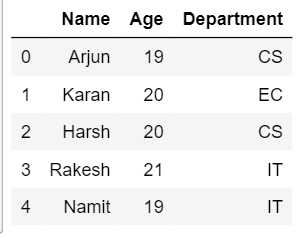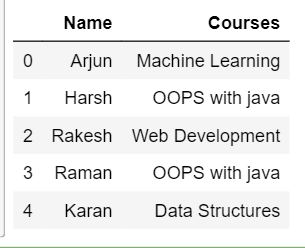#### Performing a Vlook on different types of Joins

• Inner join: Inner join produces an output data frame of only those rows for which the condition is satisfied in both the rows. To perform inner join you may specify inner as a keyword in how.

Exmaple:

 `# import pandas ` `import` `pandas as pd ` `  `  `# read csv data ` `df1 ``=` `pd.read_csv(``'Student_data.csv'``) ` `df2 ``=` `pd.read_csv(``'Course_enrolled.csv'``) ` `  `  `inner_join ``=` `pd.merge(df1,  ` `                      ``df2,  ` `                      ``on ``=``'Name'``,  ` `                      ``how ``=``'inner'``) ` `inner_join `

Output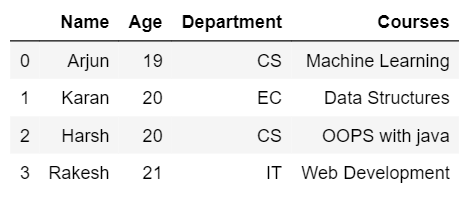• Left join: Left join operation provides all the rows from 1st dataframe and matching rows from the 2nd dataframe. If the rows are not matched in the 2nd dataframe then they will be replaced by NaN.

Example:

 `# import pandas ` `import` `pandas as pd ` `  `  `# read csv data ` `df1 ``=` `pd.read_csv(``'Student_data.csv'``) ` `df2 ``=` `pd.read_csv(``'Course_enrolled.csv'``) ` `  `  `Left_join ``=` `pd.merge(df1,  ` `                     ``df2,  ` `                     ``on ``=``'Name'``,  ` `                     ``how ``=``'left'``) ` `Left_join `

Ouput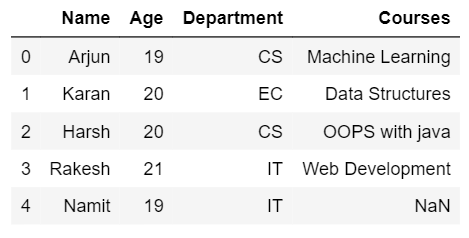• Right join: Right join is somewhat similar to left join in which the output dataframe will consist of all the rows from the 2nd dataframe and matching rows from the 1st dataframe. If the rows are not matched in 1st row then they will be replaced by NaN

 `# import pandas ` `import` `pandas as pd ` `  `  `# read csv data ` `df1 ``=` `pd.read_csv(``'Student_data.csv'``) ` `df2 ``=` `pd.read_csv(``'Course_enrolled.csv'``) ` `  `  `Right_join ``=` `pd.merge(df1,  ` `                      ``df2,  ` `                      ``on ``=``'Name'``, ` `                      ``how ``=``'right'``) ` `Right_join `

Output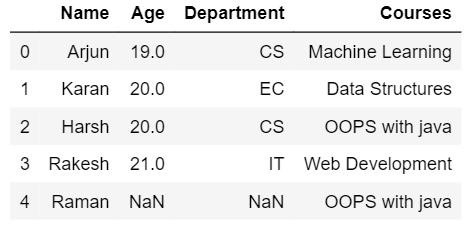• Outer join: Outer join provides the output dataframe consisting of rows from both the dataframes. Values will be shown if rows are matched otherwise NaN will be shown for rows that do not match.

Example:

 `# import pandas ` `import` `pandas as pd ` `  `  `# read csv data ` `df1 ``=` `pd.read_csv(``'Student_data.csv'``) ` `df2 ``=` `pd.read_csv(``'Course_enrolled.csv'``) ` `  `  `Outer_join ``=` `pd.merge(df1,  ` `                      ``df2,  ` `                      ``on ``=``'Name'``,  ` `                      ``how ``=``'outer'``) ` `Outer_join `

Output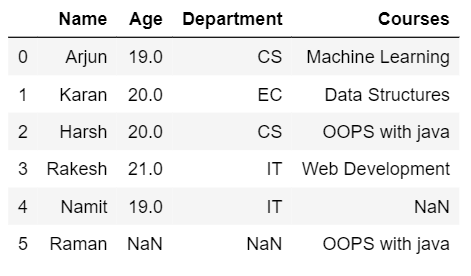My Personal Notes arrow_drop_upCheck out this Author's contributed articles.

If you like GeeksforGeeks and would like to contribute, you can also write an article using contribute.geeksforgeeks.org or mail your article to contribute@geeksforgeeks.org. See your article appearing on the GeeksforGeeks main page and help other Geeks.

Please Improve this article if you find anything incorrect by clicking on the "Improve Article" button below.

Article Tags :

1

Please write to us at contribute@geeksforgeeks.org to report any issue with the above content.